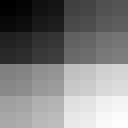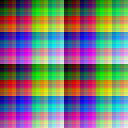# Z Curve

The Z curve is a line which zig-zags through a space, going through all points in a particular order. It’s a nice, quick way to enumerate points in a way which sort-of preserves locality, or ‘closeness’, between points in the space and points on the resulting line. Its locality isn’t as good as the Hilbert curve’s, but the Z curve is embarassingly simple to understand:

Given a point with coordinates `(x, y)`, we can find out its position on the Z curve by writing `x` and `y` in binary, interleaving their bits, then reading back the resulting number.

For example, the point `(` `5` `,` `10` `)` can be written in binary as `(` `0101` `,` `1010` `)`. If we interleave these, we get `0` `1` `1` `0` `0` `1` `1` `0`, which converted to decimal is `102`.

To convert back to `(x, y)` coordinates we just read the even and odd bits, respectively.

The `interleave` function will interleave lists of `Bits` whilst the `outerleave` function will extract lists of `Bits` from a single list:

``````interleave :: [Bits] -> Bits
interleave l = case l of
[]        -> []  -- No lists to interleave
[]    :_  -> []  -- We abort as soon as an empty list is spotted
(x:xs):ys -> x : interleave (ys ++ [xs]) -- Extract x, send xs to the end

outerleave :: Int -> Bits -> [Bits]
outerleave n xs = let (heads, xs') = splitAt    n xs          -- Take one bit for each list
tails        = outerleave n xs'         -- Recurse to get the rest
lists        = zipWith (:) heads tails  -- Prepend the heads to their tails
in if length xs < n
then replicate n []                  -- Base case
else lists``````

For example, we can colour our Z curve with a black-to-white gradient, then zig-zag it across a 2D space:

``f x y = adjust . fromBits \$ interleave [toBits y, toBits x]``

Since the interleaved number has twice as many bits, we must `adjust` it to fit in the ```128 ``` range we’re using:

``adjust = (`div` (2 ^ scale))``

This results in the following image:Notice that the gradient is quite smooth: large discontinuities are rare, common discontinuities are small. That’s because the curve has good locality.

## Higher Dimensions

Since the Z curve works for any number of dimensions, we can zig-zag it through the 3D RGB colour space, where the `(x, y, z)` coordinates we interleave correspond to red, green and blue colour intensities respectively.

Like before, we interleave the `x` and `y` coordinates of our pixels to get their position on the Z curve:

``f x y = rgbCube \$ interleave [toBits y, toBits x]``

To get our red, green and blue values, we just ‘outerleave’ three numbers from this position:

``````rgbCube xs = let [r, g, b] = outerleave 3 xs
``````adjust = let cubeScale = floor \$ fromIntegral (scale * 2) / 3